# How to Convert a List to a DataFrame Row in Python?

• Last Updated : 28 Dec, 2021

In this article, we will discuss how to convert a list to a dataframe row in Python.

## Method 1: Using T function

This is known as the Transpose function, this will convert the list into a row. Here each value is stored in one column.

Syntax: pandas.DataFrame(list).T

Example:

## Python3

 `# import pandas module``import` `pandas as pd` `# consider a list``list1 ``=` `[``"durga"``, ``"ramya"``, ``"meghana"``, ``"mansa"``]` `# convert the list into dataframe row``data ``=` `pd.DataFrame(list1).T` `# add columns``data.columns ``=` `[``'student1'``, ``'student2'``,``                ``'student3'``, ``'student4'``]` `# display``data`

Output: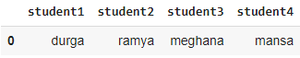## Method 2: Creating from multi-dimensional list to dataframe row

Here we are converting a list of lists to dataframe rows

Syntax: pd.DataFrame(list)

where list is the list of lists

Example:

## Python3

 `# import pandas module``import` `pandas as pd` `# consider a list``list1 ``=` `[[``"durga"``, ``"java"``, ``90``], [``"gopi"``, ``"python"``, ``80``],``         ``[``"pavani"``, ``"c/cpp"``, ``94``], [``"sravya"``, ``"html"``, ``90``]]` `# convert the list into dataframe row``data ``=` `pd.DataFrame(list1)` `# add columns``data.columns ``=` `[``'student1'``, ``'subject'``, ``'marks'``]` `# display``data`
[tabb

ing]

Output: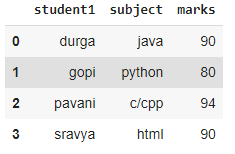## Method 3: Using a list with index and columns

Here we are getting data (rows ) from the list and assigning columns to these values from columns

Syntax: pd.DataFrame(list, columns, dtype )

where

• list is  the list of input values
• columns are the column names for list of values
• dtype is the column data type

Example:

## Python3

 `# import pandas module``import` `pandas as pd` `# consider a list``list1 ``=` `[[``"durga"``, ``"java"``, ``90``], [``"gopi"``, ``"python"``, ``80``],``         ``[``"pavani"``, ``"c/cpp"``, ``94``], [``"sravya"``, ``"html"``, ``90``]]` `# convert the list into dataframe row by adding columns``data ``=` `pd.DataFrame(list1, columns``=``[``'student1'``,``                                    ``'subject'``,``                                    ``'marks'``])`  `# display``data`

Output:## Method 4: Using zip() function

Here  we are taking separate lists as input such that each list will act as one column, so the number of lists = n columns in the dataframe, and using zip function we are combining the lists.

Syntax pd.DataFram

st(zip(list1,list2,.,list n)),columns)

where

• columns is the column for the list values
• list1.list n represent number of input lists for columns

Example:

## Python3

 `# import pandas module``import` `pandas as pd` `# consider a list``list1 ``=` `[``"durga"``, ``"ramya"``, ``"sravya"``]``list2 ``=` `[``"java"``, ``"php"``, ``"mysql"``]``list3 ``=` `[``67``, ``89``, ``65``]` `# convert the list into dataframe row by``# using zip()``data ``=` `pd.DataFrame(``list``(``zip``(list1, list2, list3)),``                    ``columns``=``[``'student'``, ``'subject'``, ``'marks'``])`  `# display``data`

Output: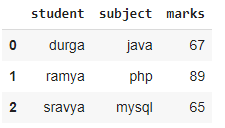## Method 5: Using a list of dictionary

Here we are passing the individual lists which act as columns in the data frame to keys to the dictionary, so by passing the dictionary into dataframe() we can convert list to dataframe.

Syntax: pd.DataFrame{‘key’: list1, ‘key’: list2, ……..,’key’: listn}

These keys will be the column names in the dataframe.

Example:

"noIdeBtnDiv">

## Python3

 `# import pandas module``import` `pandas as pd` `# consider a list``list1 ``=` `[``"durga"``, ``"ramya"``, ``"sravya"``]` `list2 ``=` `[``"java"``, ``"php"``, ``"mysql"``]` `list3 ``=` `[``67``, ``89``, ``65``]` `# convert the list into dataframe row by``# using dictionary``dictionary ``=` `{``'name'``: list1, ``'subject'``: list2,``              ``'marks'``: list3}` `data ``=` `pd.DataFrame(dictionary)` `# display``data`

Output: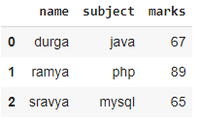## Method 6: Creating from  multi-dimensional list to dataframe row with columns

Here we are taking input from multi-dimensional lists and assigning column names in the DataFrame() function

Syntax: pd.DataFrame(list,columns)

where

1. list is an multidimensional list
2. columns are the column names

Example:

## Python3

 `# import pandas module``import` `pandas as pd` `# consider a list``list1 ``=` `[[``"durga"``, ``"java"``, ``90``],``         ``[``"gopi"``, ``"python"``, ``80``],``         ``[``"pavani"``, ``"c/cpp"``, ``94``],``         ``[``"sravya"``, ``"html"``, ``90``]]` `# convert the list into dataframe``# row using columns from multi lists``data ``=` `pd.DataFrame(list1, columns``=``[``'student1'``,``                                    ``'subject'``,``                                    ``'marks'``])` `# display``data`

Output: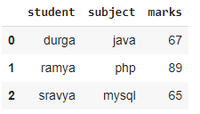My Personal Notes arrow_drop_up